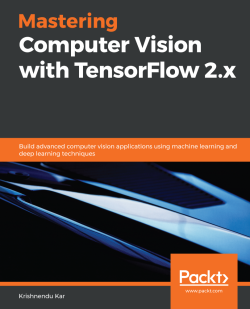### Mastering Computer Vision with TensorFlow 2.x

By Krishnendu Kar
• Constantly updated with 100+ new titles each month
• Breadth and depth in over 1,000+ technologies

Publication date:
May 2020
Publisher
Packt
Pages
430
ISBN
9781838827069

# Section 1: Introduction to Computer Vision and Neural Networks

In this section, you will develop your understanding of the theory as well as learn hands-on techniques about the application of a convolutional neural network for image processing. You will learn key concepts such as image filtering, feature maps, edge detection, the convolutional operation, activation functions, and the use of fully connected and softmax layers in relation to image classification and object detection. The chapters provide many hands-on examples of an end-to-end computer vision pipeline using TensorFlow, Keras, and OpenCV. The most important learning that you will take from these chapters is to develop an understanding and intuition behind different convolutional operations – how images are transformed through different layers of convolutional neural networks.

By the end of this section, you will be able to do the following:

• Understand how image filters transform an image (chapter 1)
• Apply various types of image filters for edge detection (chapter 1)
• Detect simple objects using OpenCV contour detection and Histogram of Oriented Gradients (HOG) (Chapter 1)
• Find the similarity between objects using Scale-invariant feature transform (SIFT), Local Binary Patterns (LBP) pattern matching, and color matching (chapters 1 and 2)
• Face detection using the OpenCV cascade detector (chapter 3)
• Input big data into a neural network from a CSV file list and parse the data to recognize columns, which can then be fed to the neural network as x and y values (chapter 3)
• Facial keypoint and facial expression recognition (chapter 3)
• Develop an annotation file for facial keypoints (chapter 3)
• Input big data into a neural network from files using the Keras data generator method (chapter 4)
• Construct your own neural network and optimize its parameters to improve accuracy (chapter 4)
• Write code to transform an image through different layers of the convolutional neural network (chapter 4)

This section comprises the following chapters:

• Chapter 1Computer Vision and TensorFlow Fundamentals
• Chapter 2Content Recognition Using Local Binary Pattern
• Chapter 3Facial Detection Using OpenCV and CNN
• Chapter 4Deep Learning on Images